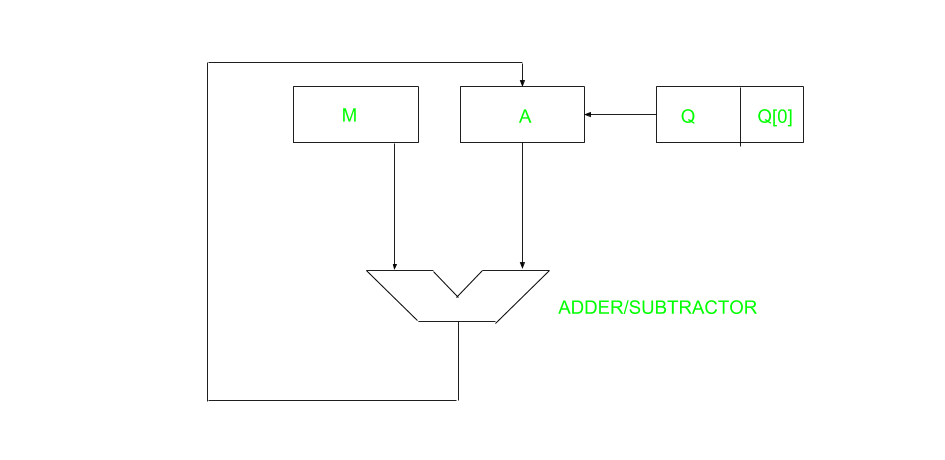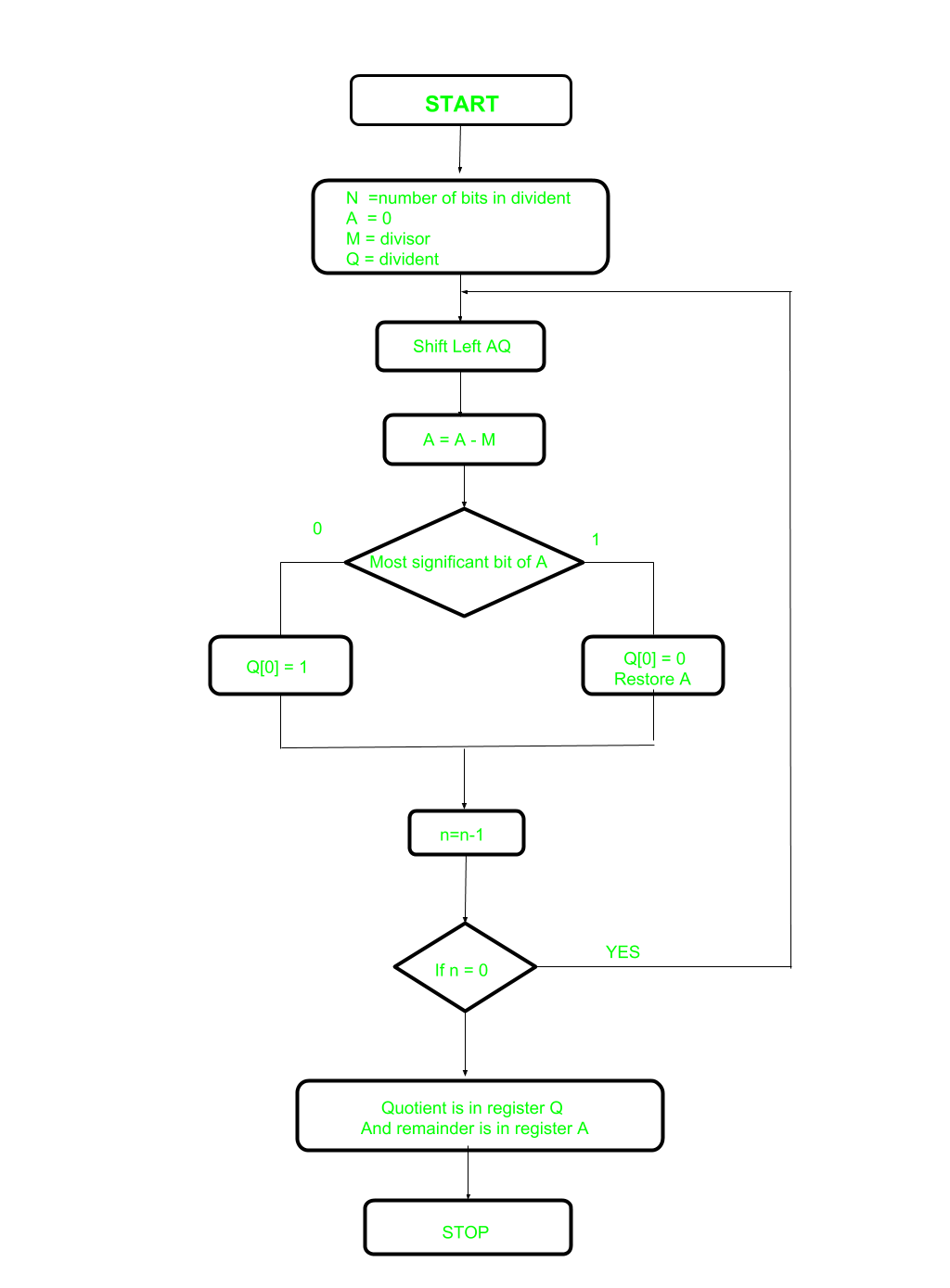# Restoring Division Algorithm For Unsigned Integer

A division algorithm provides a quotient and a remainder when we divide two number. They are generally of two type slow algorithm and fast algorithm. Slow division algorithm are restoring, non-restoring, non-performing restoring, SRT algorithm and under fast comes Newton–Raphson and Goldschmidt.

In this article, will be performing restoring algorithm for unsigned integer. Restoring term is due to fact that value of register A is restored after each iteration.Here, register Q contain quotient and register A contain remainder. Here, n-bit dividend is loaded in Q and divisor is loaded in M. Value of Register is initially kept 0 and this is the register whose value is restored during iteration due to which it is named Restoring.Let’s pick the step involved:

• Step-1: First the registers are initialized with corresponding values (Q = Dividend, M = Divisor, A = 0, n = number of bits in dividend)
• Step-2: Then the content of register A and Q is shifted right as if they are a single unit
• Step-3: Then content of register M is subtracted from A and result is stored in A
• Step-4: Then the most significant bit of the A is checked if it is 0 the least significant bit of Q is set to 1 otherwise if it is 1 the least significant bit of Q is set to 0 and value of register A is restored i.e the value of A before the subtraction with M
• Step-5: The value of counter n is decremented
• Step-6: If the value of n becomes zero we get of the loop otherwise we repeat from step 2
• Step-7: Finally, the register Q contain the quotient and A contain remainder

Examples:

```Perform Division Restoring Algorithm
Dividend = 11
Divisor  = 3```
n M A Q Operation
4 00011 00000 1011 initialize
00011 00001 011_ shift left AQ
00011 11110 011_ A=A-M
00011 00001 0110 Q=0 And restore A
3 00011 00010 110_ shift left AQ
00011 11111 110_ A=A-M
00011 00010 1100 Q=0
2 00011 00101 100_ shift left AQ
00011 00010 100_ A=A-M
00011 00010 1001 Q=1
1 00011 00101 001_ shift left AQ
00011 00010 001_ A=A-M
00011 00010 0011 Q=1

Remember to restore the value of A most significant bit of A is 1. As that register Q contain the quotient, i.e. 3 and register A contain remainder 2.My Personal Notes arrow_drop_upIf you like GeeksforGeeks and would like to contribute, you can also write an article using contribute.geeksforgeeks.org or mail your article to contribute@geeksforgeeks.org. See your article appearing on the GeeksforGeeks main page and help other Geeks.

Please Improve this article if you find anything incorrect by clicking on the "Improve Article" button below.

Improved By : ShubhamMaurya3

1

Please write to us at contribute@geeksforgeeks.org to report any issue with the above content.